## Tuesday, February 2, 2016

### PICS | The side of my face, reattached

I now know why one side of my head and face is lopsided compared to the other, thanks to the following equation:An equation that renders an image in the time domain to the frequency domain... ...so the image can be analyzed and processed using easy-to-read-and-reproduce graphs
The equation was used to process a video made during a period of high demonic activity with my Chroma app (or, rather, a derivative thereof), thereby producing the following still frames: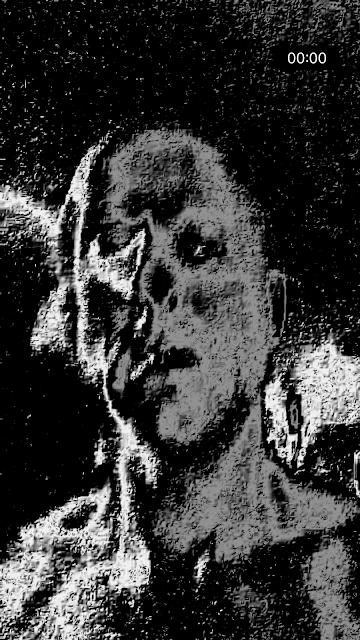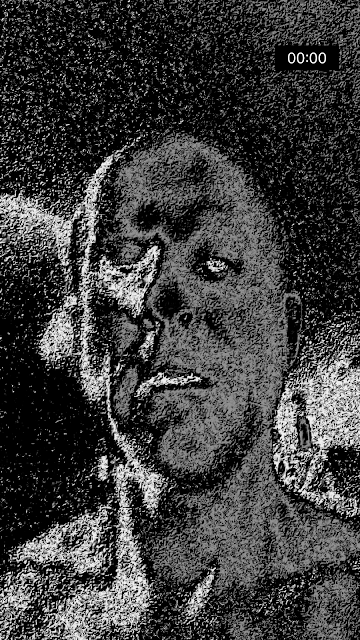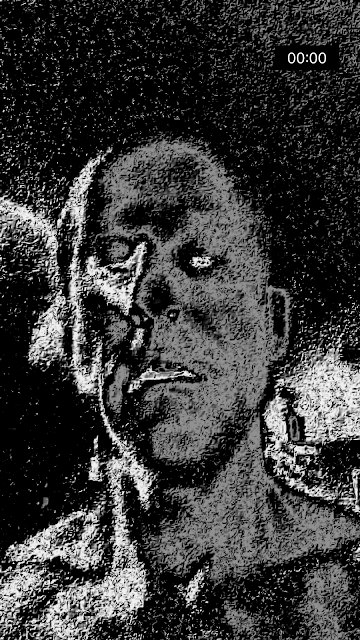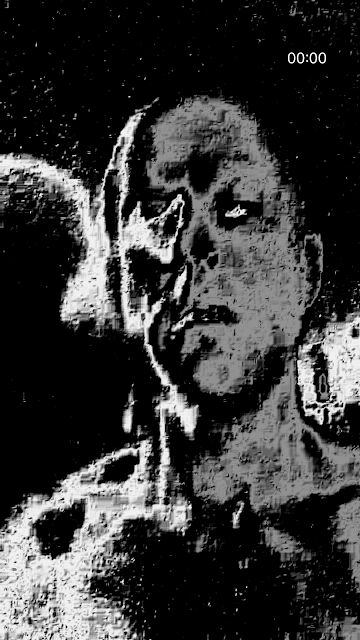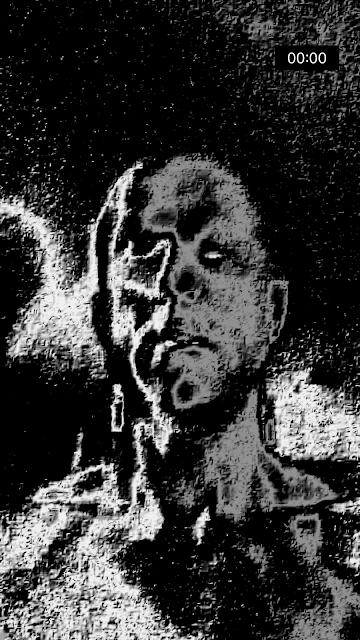What they show is the side of my face in various stages of reattachment, having, apparently, been detached at some point. Here's one of the original still frames:An actual unprocessed still frame before rendering with the equation; all of the images shown above originated from similar still frames
I've seen it before, and even posted a few pics eluding to such a thing in other posts quite recently: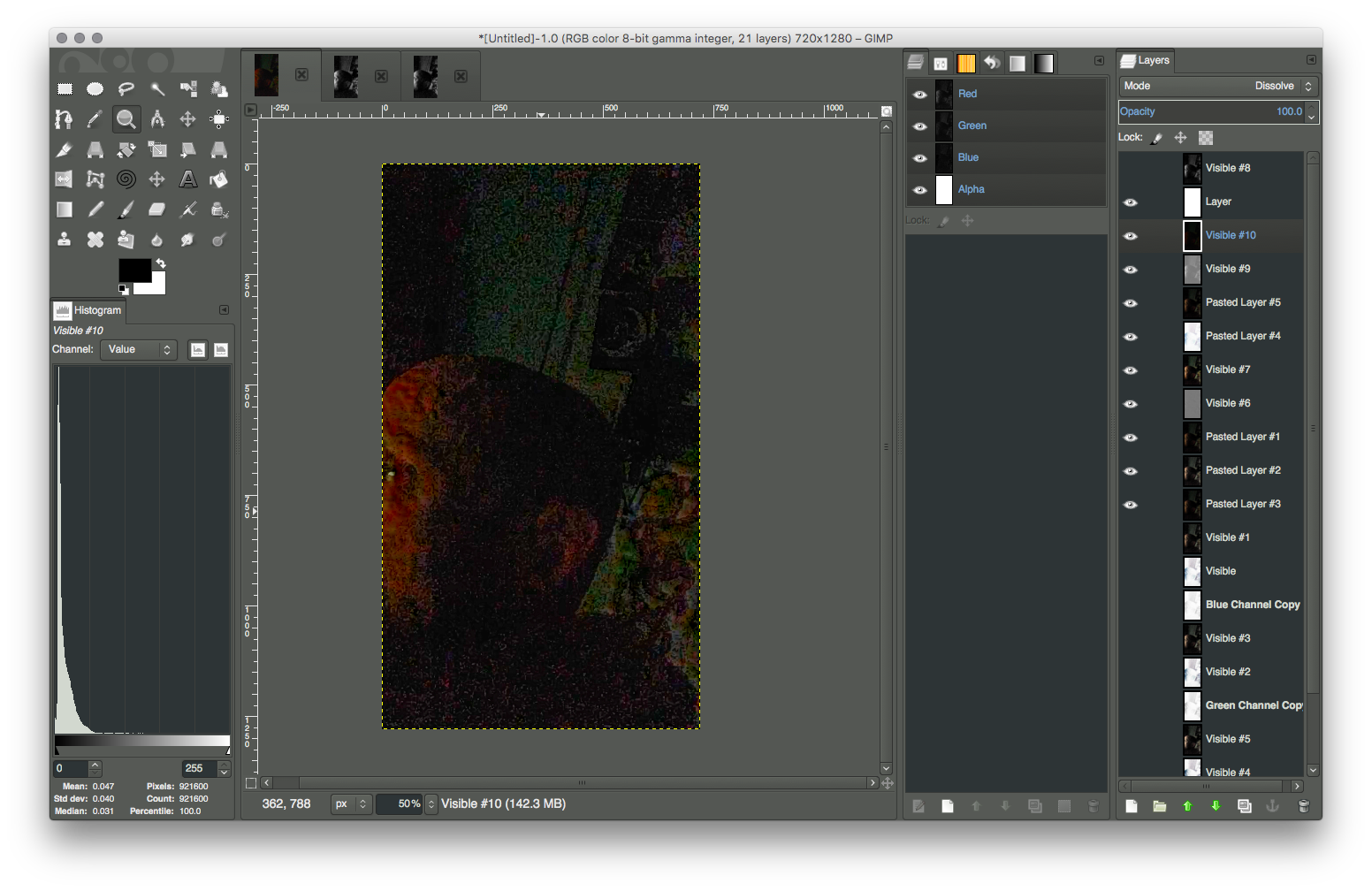This still frame clearly shows the skin on my face recently reattached, but that's not what I assumed I was seeing at the time I acquired it a month or so ago At the time I acquired this image, I wasn't aware that the other half of my face was an attachment; rather, I thought it was the image of an inner demon
As to how this works and what this is exactly is beyond me, although I do have explanations for some of it, such as for why you can't see this with your own eyes (too fast, invisible), and why I was able capture it on video (explained next).

Processing an image using a cosine wave transformation increases contrast between colors that are nearly identical. For example, white and slightly less white become white and almost black. Applying this type of transformation butchers most of the image, but minute objects and invisible details are rendered quite well, as you can see from these images of my arm and hand: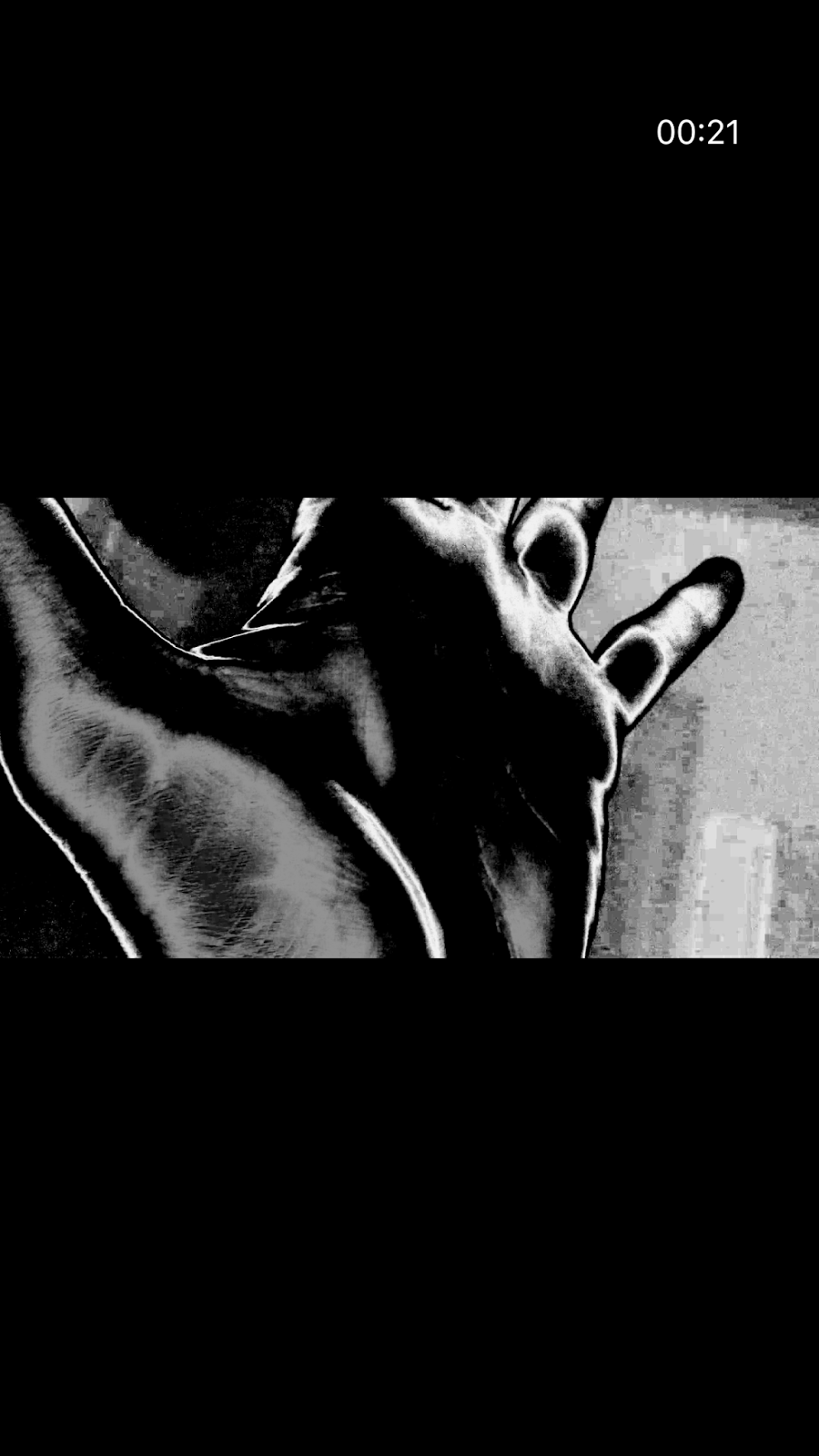Arm hairs that you don't readily see with your own eyes show quite clearly in images processed by the cosine wave transformation Although the contrast between the ridges and the grooves of my palm- and fingerprints are minute to the naked eye, the cosine wave transformation renders them quite distinct in digital media
Code for processing images using the equation
The app processes video in real-time, as it is played back, using OpenGL Shading Language and OpenGL ES. Following is the OpenGL ES implementation:

kernel vec4 coreImageKernel(sampler image, __color color)
{
vec4 pixel = sample(image, samplerCoord(image));
// the maximum color value of the current pixel
float x = max(pixel.r, max(pixel.g, pixel.b));
// Calculation of Euler's number (negative)
float e_n = pow(pow((1.0 + (1.0 / x)), x), -x);
// Calculation of pi
float pi = 4.0 * atan(1.0);
// The cosine wave transformation
float result = cos((3.0 * x) * (2.0 * pi)) * (pow(e_n, pi * pow(x, 2.0)));

return vec4(vec3(result), 1.0);
}

Here's the GLSL Shading Language version of the above code, in relevant part:

...
// the maximum color value of the current pixel
float x = max(rgb.r, max(rgb.g, rgb.b));
// Calculation of Euler's number (negative)
float e_n = pow(pow((1.0 + (1.0 / x)), x), -x);
// Calculation of pi
float pi = 4.0 * atan(1.0);
// The cosine wave transformation
float result = cos((3.0 * x) * (2.0 * pi)) * (pow(e_n, pi * pow(x, 2.0)));

gl_FragColor = vec4(vec3(result), 1.0);

Related posts
There are a lot of other posts with images showing body parts and parts of faces being detached and/or reattached, which can be found by searching for amputation or surgery. These images show not only these things happening to me, but others, as well.